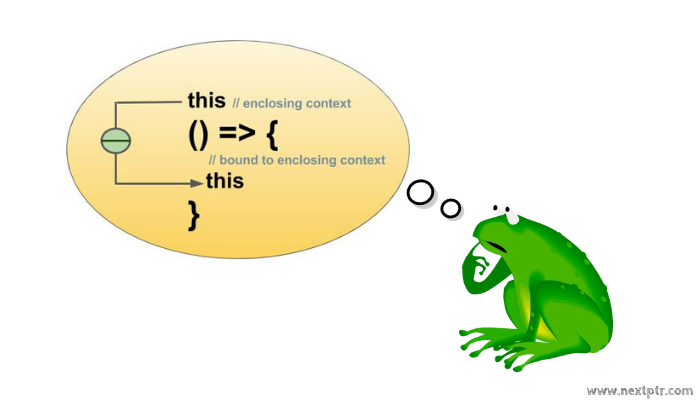# The 'this' is bound to enclosing context in a JavaScript Arrow functionIf you also have to deal with all the nuisance of capturing the this for enclosed functions, then Arrow Functions is easily going to be your most favorite JavaScript ES6 addition. Arrow functions have two salient features:

1. A shorter syntax for anonymous functions or function expressions. `(x,y) => x+y;` for `function (x,y) { return x+y; }`
2. The 'this' in an Arrow function is captured from enclosing lexical scope.

We are going to talk about the second feature in this post. Here is an example code with regular function expression:

``````var student = {

courses: ['Linear Algebra',
'Data Structures',
'Operating Systems'],

grades:  [ 4.0, 3.0, 3.5 ],

// Get the max grade course
var self = this;  // Capture 'this'
};
}
};
``````

Notice how we captured the this in getMaxGradeCourse() into self for enclosed function expression maxGrade. The above implementation of maxGrade with Arrow function would be:

``````getMaxGradeCourse: function() {
}
``````

The Arrow function has captured the this from enclosing lexical scope, which is bound to enclosing context object student. Not only it is easier to understand but also quite terse.

At this point I must emphasize that if the Arrow function expression is a property of an object, you should not assume the this inside the Arrow function is bound to that object. Once Again,

this in an Arrow function is bound to enclosing context.

Keep that in mind and you are going to answer below question correct.

Suppose we add another method property - isCourseTaken - to student:

``````var student = {
courses: ['Linear Algebra',
'Data Structures',
'Operating Systems'],
//....more properties....
isCourseTaken: (c) => {
return this.courses.indexOf(c) != -1;
}
};

console.log( student.isCourseTaken('Linear Algebra') );
``````

What will be the outcome of above?